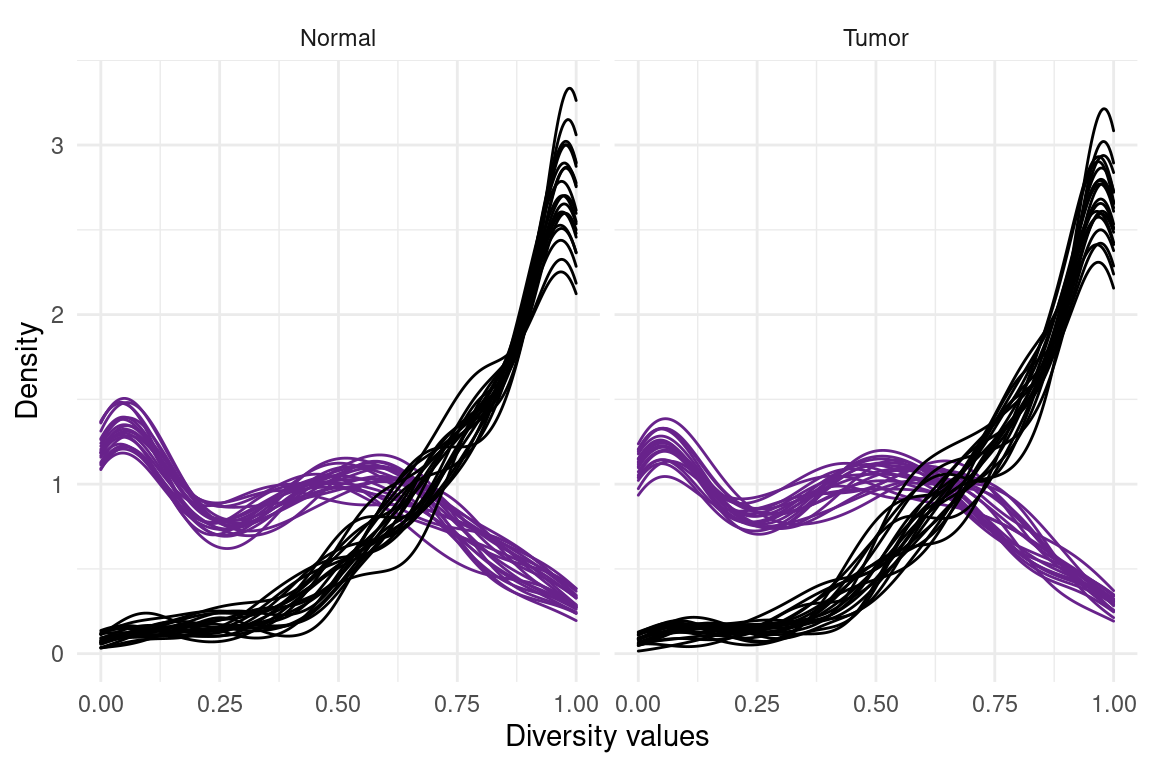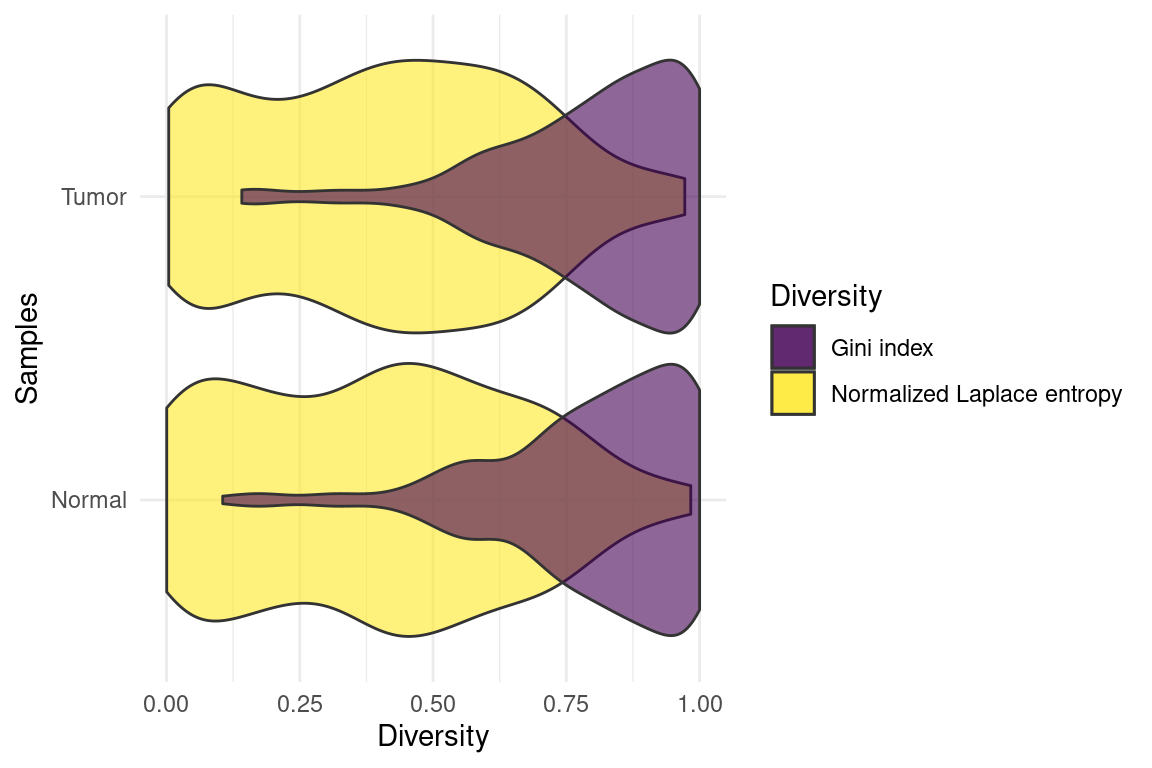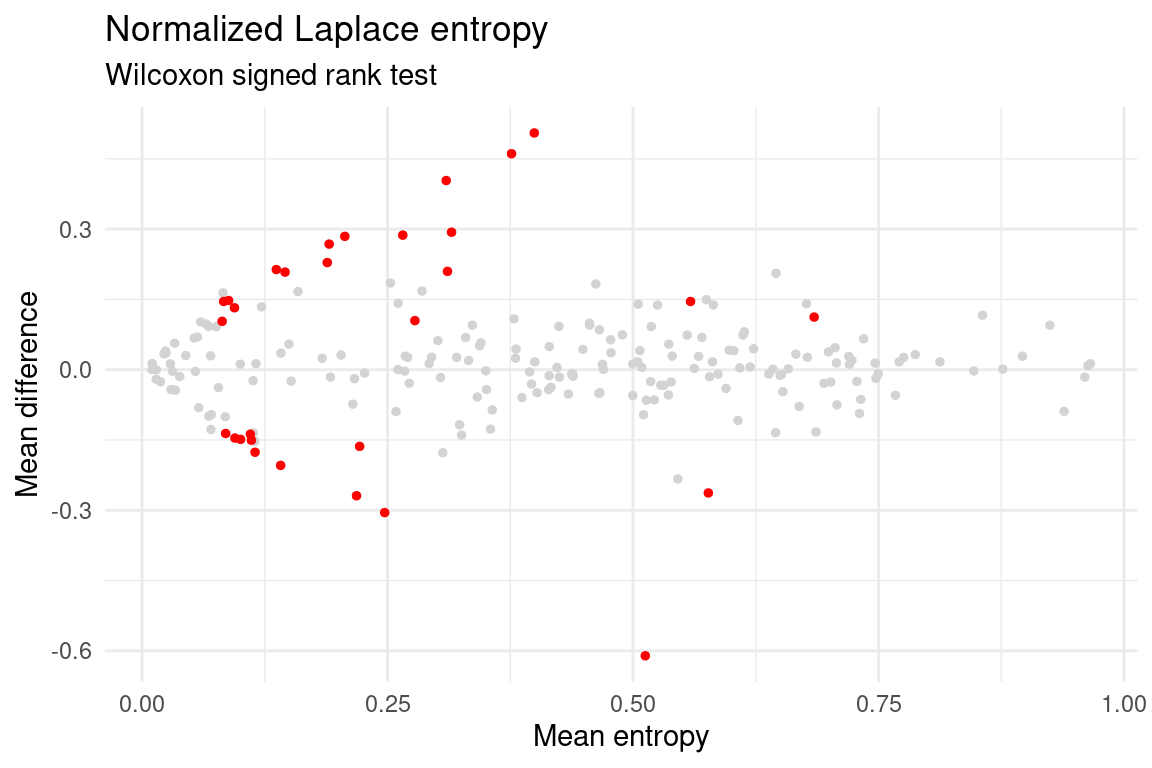Standard workflow

SplicingFactory package version: 1.2.0

Input data structure

As input, the SplicingFactory package can use 5 different data types. Besides matrices and data frames, you can use the output of tximport, DGELists and SummarizedExperiments. The tximport R package is able to import files containing transcript abundance estimates generated by tools such as Salmon, Kallisto or RSEM. The tximport datasets are stored in a list by default, and they can serve as input for SplicingFactory package.

DGELists are a list-based S4 class for storing read counts and associated information, while SummarizedExperiment objects contain and describe high-throughput expression level assays. SplicingFactory automatically uses the necessary functions for all of these data types, on condition that they were not previously modified (e.g. list elements renamed).

While SplicingFactory can process any kind of numeric value, used to measure expression levels, we recommend TPM or similar length normalized values. If the read count values are not normalized for the transcript isoform lengths, the read count proportions, therefore the diversity values, will be misleading. For example, a gene with three transcript isoforms, lengths of 100, 100, and 1000, and read counts of 20, 20 and 200 for each of them is detected in an experiment. Simply using the read counts to calculate proportions, will lead to the values of 0.083, 0.083 and 0.83, and to the conclusion that we have a single dominant isoform based on the diversity value. However,the third isoform is 10 times longer than the other two, leading to a larger number of reads originating from this isoform. Normalizing for isoform length will lead to the same 0.33 proportion for all of them, therefore no dominant isoform and a very different diversity value.

Data arrangement

Besides the format, the input table (matrix, data.frame or the tabular expression data extracted from other data structures) needs to be arranged properly. Every row identifies a distinct transcript in your dataset, while every column belongs to a distinct sample. The table can contain only numeric values. Supplementing your table, the package will need a gene and a sample vector, identifying the genes and the sample conditions in your analysis. The gene vector assigns genes to every row in your data, that will be used to aggregate transcript level expression information at the gene level. It is important that the columns and the rows of the table (genes and samples) are in the same order, as the gene and the sample vectors. In case of SummarizedExperiment, the genes vector can be empty (but not exclusively) as the package can automatically extract the necessary vector from the object.

Example dataset

The package contains an example dataset called tcga_brca_luma_dataset. The data was downloaded from The Cancer Genome Atlas (TCGA) on 12th of April, 2020. It contains transcript level read counts for 300 pre-selected genes of 40 patients with Luminal A type breast cancer (primary tumor and solid normal samples). Transcript level expression was estimated with RSEM.

You can check the list of TCGA sample ids selected with the following code.

You can check the list of pre-selected genes with the following code.

Splicing diversity analysis

Data filtering and preprocessing

As a first step, before doing the diversity calculation, you might want to filter out genes with a low overall expression or limit the analysis to transcripts with a sufficient minimum expression level. Expression estimates of transcript isoforms with zero or low expression might be highly variable. For more details on the effect of transcript isoform prefiltering on differential transcript usage, see this paper.

Here, we are filtering out transcript isoforms with less than 5 reads in more than 5 samples. Additionally, we update the genes vector to match the new filtered matrix.

Transcript diversity calculation

We are going to use the calculate_diversity function to calculate two different types of transcript diversity.There are several mandatory and optional pamaters for the function. Even though we are using only a limited number of genes for a set of 40 samples, the analysis can be done using a full transcriptome annotation and much larger sample sets.

• x - Input data, in various formats, discussed in more detail in the Input data structure and Data arrangement section.
• genes - A vector with gene names used for aggregating the transcript level data.
• method - Method to use for splicing diversity calculation.
• norm - If set to TRUE, the entropy values are normalized to the number of transcripts for each gene.
• tpm - In the case of a tximport list, you might want set the tpm argument. As the default option is FALSE, the raw read counts will be extracted from your input data. Set it to TRUE if you want to use TPM values.
• assayno - An optional argument is assayno, which is useful if you are planning to analyze a SummarizedExperiment input, containing multiple assays. assayno is a numeric value, specifying the assay to be analyzed.
• verbose - Set it to TRUE if you want more detailed diagnostic messages.

To calculate Laplace entropy, where values are normalized between 0 and 1, use:

To calculate Gini index, you don’t need to specify the norm argument, as the Gini index is by definition ranges between 0 (complete equality) and 1 (complete inequality).

Both for the Laplace-entropy and Gini index calculation, the package returns a SummarizedExperiment object, that you can investigate further with the assay function.

The package automatically filters out genes with a single isoform, as splicing diversity values can only be calculated for genes with at least 2 splicing isoforms.

Some genes might show NA diversity values. This means that the expression was zero for all transcript isoforms of the gene in these samples and the package could not calculate any diversity value as there is no meaningful diversity for genes which did not show any expression in your experiment. Lack of expression might also be the result of technical issues.

To further analyze and visualize your data, you might do the following. To see the distribution and density of the splicing diversity data, you can visualize it by using ggplot2 from the tidyverse package collection.

library("tidyr")
#>
#> Attaching package: 'tidyr'
#> The following object is masked from 'package:S4Vectors':
#>
#>     expand
library("ggplot2")

# Construct data.frame from SummarizedExperiment result
laplace_data <- cbind(assay(laplace_entropy),
Gene = rowData(laplace_entropy)\$genes)

# Reshape data.frame
laplace_data <- pivot_longer(laplace_data, -Gene, names_to = "sample",
values_to = "entropy")

laplace_data\$sample_type <- apply(laplace_data[, 2], 1,
function(x) ifelse(grepl("_N", x),
"Normal", "Tumor"))

# Filter genes with NA entropy values
laplace_data <- drop_na(laplace_data)

# Update gene names and add diversity type column
laplace_data\$Gene <- paste0(laplace_data\$Gene, "_", laplace_data\$sample_type)
laplace_data\$diversity <-  "Normalized Laplace entropy"

# Construct data.frame from SummarizedExperiment result
gini_data <- cbind(assay(gini_index), Gene = rowData(gini_index)\$genes)

# Reshape data.frame
gini_data <- pivot_longer(gini_data, -Gene, names_to = "sample",
values_to = "gini")

gini_data\$sample_type <- apply(gini_data[, 2], 1,
function(x) ifelse(grepl("_N", x),
"Normal", "Tumor"))

# Filter genes with NA gini values
gini_data <- drop_na(gini_data)

# Update gene names and add diversity type column
gini_data\$Gene <- paste0(gini_data\$Gene, "_", gini_data\$sample_type)
gini_data\$diversity <-  "Gini index"

# Plot diversity data
ggplot() +
geom_density(data = laplace_data, alpha = 0.3,
aes(x = entropy, group = sample, color = diversity)) +
geom_density(data = gini_data, alpha = 0.3,
aes(x = gini, group = sample, color = diversity)) +
facet_grid(. ~ sample_type) +
scale_color_manual(values = c("black", "darkorchid4")) +
guides(color = FALSE) +
theme_minimal() +
labs(x = "Diversity values",
y = "Density")
#> Warning: `guides(<scale> = FALSE)` is deprecated. Please use `guides(<scale> =The two methods are calculating different results. Genes with a single dominant isoform have a near-zero entropy, while they have a Gini index close to 1. The overall distribution of the data is similar between the Normal and Tumor conditions.

Differential analysis

To further analyze the data, the steps of a differential diversity analysis are implemented in the calculate_difference function, aiming to identify genes with significant changes in splicing diversity. The result table will contain the mean or median values of the diversity across sample categories, the difference of these values, the log2 fold change of the two different conditions, p-values and adjusted p-values for each genes.

There are several mandatory and optional arguments for this function:

• x - Output of the previous function, which is a data.frame with gene names as the first column and splicing diversity values for each sample in additional columns.
• samples - The previously defined vector with sample conditions specifying the category of each sample in the correct order.
• control - Name of the control sample category, defined in the samples vector, e.g. control = “Normal” or control = “WT”.
• method - Method to use for calculating the average splicing diversity value in a condition. Can be “mean” or “median”.
• test - Method to use for p-value calculation. There are two statistical tests implemented at the moment - Wilcoxon rank sum test and label shuffling test.
• randomizations - Optional parameter for the label shuffling test. You can specify the number of random shuffles (default = 100).
• pcorr - P-value correction method to use for the test results. Benjamini & Hochberg by default.
• assayno - An optional argument is assayno, which is useful if you are planning to analyze a SummarizedExperiment input, containing multiple assays. assayno is a numeric value, specifying the assay to be analyzed.
• verbose - Set it to TRUE if you want more detailed diagnostic messages.

To analyze the previously calculated normalized Laplace entropy values stored in a SummarizedExperiment object with a Wilcoxon sum rank test, use the calculate_difference function as follows:

The package sends a note about 11 genes, with low sample size, excluded from the analysis. as these genes had several NA diversity values, the result of 0 expression values. You need at least 3 samples in each sample category and a total of 8 samples for a Wilcoxon test and at least 5 samples in each sample category for the label shuffling.

Genes with a significant change in splicing diversity can be further analyzed and visualized by using e.g. MA-plots, where the log2 fold change or mean difference is shown on the y axis, and the mean diversity values on the x axis. Dots are colored red if the adjusted p-value is smaller than 0.05. It is recommended to filter for an absolute mean difference larger than 0.1 besides the adjusted p-value.

The normalized naive and Laplace entropy, the Gini index, and the Simpson index are bounded in [0, 1], and we recommend using the mean or median difference when filtering for biologically meaningful changes. The non-normalized naive and Laplace entropy and the inverse Simpson index are not bounded in [0, 1], and the log2 fold change might give more useful results.The analyzed genes also can be visualized on a Volcano-plot, which shows the adjusted p-values of the genes on a logarithmic scale on the y axis and the mean difference values between the two conditions on the x axis. We used a cutoff of 0.1 and -0.1 for the mean difference.